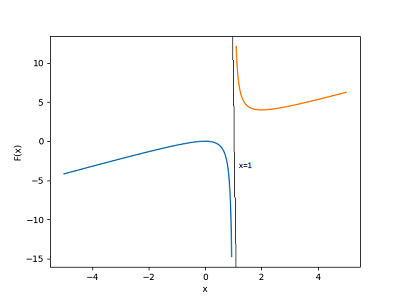# Sketch the graph of F(x)=\frac{x^2}{x-1} . Identify asymptotes, extrema, points of inflection...

## Question:

Sketch the graph of

{eq}\displaystyle F(x)=\frac{x^2}{x-1} {/eq}.

Identify asymptotes, extrema, points of inflection and / or axis intercepts.

## Graphing and Analysis of Rational Function:

We are given a rational function. First, we sketch a graph of this rational function. Then, using the graph as well as analytical techniques, we find horizontal and vertical asymptotes of this graph if any. Using first and second derivatives from calculus, we find the local extrema (maxima or minima) as well as points of inflection. Finally, we list any x-intercepts and/or any y-intercepts the graph may have.

(a) Below is a sketch of the graph of this function plotted using the PYTHON programming language package/module MATPLOTLIB:(b) Owing to the function being of rational form, we may note that x = 1 makes the denominator zero and hence causes the function to be undefined. In order words, as may be seen from the graph, x = 1 is a vertical asymptote.

Since the function goes to infinity for large values of x, there are no horizontal asymptotes.

(c) To find points of relative extrema, we seek critical points of F(x). To that end, we calculate the first derivative of F(x) which is as follows:

{eq}\displaystyle F'(x)=\frac {(x-1)(2x)-x^2(1)}{(x-1)^2} = \frac {x^2-2x}{(x-1)^2} \qquad (1) {/eq}

From (1) F'(x) is not defined at x = 1. However, the function is not defined at x = 1, and the limit as x approaches 1 does not exist either. So we disregard x = 1 as a critical point. Next, we find critical points x for F'(x) = 0, which from (1) gives us

{eq}x^2-2x=0 \implies x(x-2)=0 \implies x = 0, \; x = 2. {/eq}

To determine whether x = 0, 2 are relative maxima or local minima or neither we use the second derivative test. To that end, we need to calculate the second derivative of F(x), which is as below:

{eq}\displaystyle F''(x)=\frac {(x-1)^2(2x-2)-2(x-1)(x^2-2x)}{(x-1)^4} \qquad (2) {/eq}

From (2), {eq}F''(0)<0 \enspace and \enspace F''(2) > 0. {/eq}

Hence from the second derivative test, x = 0 is a point of local maximum and x = 2 is a point of local minimum. The graph above confirms these!

(d) To find points of inflection we solve for the second derivative from (2) equal to zero to get

{eq}\displaystyle \frac {(x-1)^2(2x-2)-2(x-1)(x^2-2x)}{(x-1)^4} = 0 \implies \frac {2(x-1)}{(x-1)^4}=0 \implies x = 1. {/eq}

But x = 1 cannot be a solution of {eq}F''(x)=0 {/eq} owing to division by zero. Hence there are no inflection points for F(x). However, we do note from the graph above that even though F(x) is not continuous at x = 1, the concavity of the function does change at x = 1, going from concave down to concave up.

(e) The only intercept is the origin (0, 0).